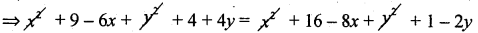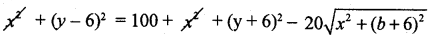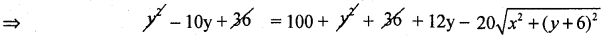# 1st PUC Basic Maths Question Bank Chapter 16 Locus and its Equations

Students can Download Basic Maths Chapter 16 Locus and its Equations Questions and Answers, Notes Pdf, 1st PUC Basic Maths Question Bank with Answers helps you to revise the complete Karnataka State Board Syllabus and score more marks in your examinations.

## Karnataka 1st PUC Basic Maths Question Bank Chapter 16 Locus and its Equations

Question 1.
Find the equation of the locus of a point which moves such that its distance from the point (-5,7) is twice its distance from (-3,1).
Let A = (-5, 7) and B = (-3, 1) and P(y, b) be any point of the locus
By data PA = 2PB = PA2 = 4PB2
⇒ (x + 5)5 + (y – 7)2
= 4[(x + 3)2 + (y – 1)2]
⇒ x2 + 10x + 25 + y2 + 49 – 14y
= 4(x2 + 9 + 6x + y2 + 1 – 2y)
= 4x2 + 36 + 24x + 4y2 + 4 – 8y
= 3x2 + 3y2 + 14x + 6y – 34 = 0
which is the equation as to the locus.

Question 2.
Find the equation to the locus of the perpendicular bisector of the line joining A(3, 2) and B(4,1).
The perpendicular bisector of the line joining A and B is the locus of the point which moves such that it is equidistant from A and B.
By data we have A = (3, -2), B = (4, 1)
Let P(x, y) be any point on the perpendicular bisector
Thus we have PA = PB = PA2 = PB2
=> (x – 3)2 + (y + 2)2 = (x – 4)2 + (y – 1 )2⇒ -6x + 4y + 13
= -8x – 2y + 17
⇒ 2x + 6y – 4 = 0.
⇒ x + 3y – 2 = 0 is the equation of the locus of a point.Question 3.
Find the equation of the locus of the point which moves such that the sum of its distances from (0, 5) and (0, -4) is 10 units.
Let A = (0, 6) and B = (0, -6).
Let P(x, y) be the locus of the point; By data PA + PB = 10
⇒ $$\sqrt{x^{2}+(y-6)^{2}}+\sqrt{x^{2}+(y+6)^{2}}$$ = 0
⇒ $$\sqrt{x^{2}+(y-6)^{2}}$$ = 10 – $$\sqrt{x^{2}+(y+6)^{2}}$$
Squaring both sides⇒ 10y – 12y – 100 = -20$$\sqrt{x^{2}+(y+6)^{2}}$$
⇒ -22y + – 100 = -20$$\sqrt{x^{2}+(y+6)^{2}}$$ + by – 2
⇒ 11y + 50 = 10$$\sqrt{x^{2}+(y+6)^{2}}$$
squaring again we get
⇒ 121y2 + 2500 + 110y
= 100(x2 + y2 + 36 + 12y)
= 100x2 + 10y2 + 3600 + 122y
⇒ 100x2 – 21y2 + 100y + 1100 = 0 is the locus of the equation.

error: Content is protected !!